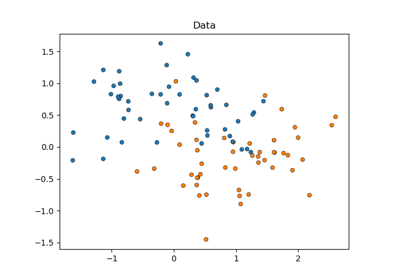# sklearn.model_selection.RepeatedStratifiedKFold¶

class sklearn.model_selection.RepeatedStratifiedKFold(*, n_splits=5, n_repeats=10, random_state=None)[source]

Repeated Stratified K-Fold cross validator.

Repeats Stratified K-Fold n times with different randomization in each repetition.

Read more in the User Guide.

Parameters:
n_splitsint, default=5

Number of folds. Must be at least 2.

n_repeatsint, default=10

Number of times cross-validator needs to be repeated.

random_stateint, RandomState instance or None, default=None

Controls the generation of the random states for each repetition. Pass an int for reproducible output across multiple function calls. See Glossary.

RepeatedKFold

Repeats K-Fold n times.

Notes

Randomized CV splitters may return different results for each call of split. You can make the results identical by setting random_state to an integer.

Examples

>>> import numpy as np
>>> from sklearn.model_selection import RepeatedStratifiedKFold
>>> X = np.array([[1, 2], [3, 4], [1, 2], [3, 4]])
>>> y = np.array([0, 0, 1, 1])
>>> rskf = RepeatedStratifiedKFold(n_splits=2, n_repeats=2,
...     random_state=36851234)
>>> rskf.get_n_splits(X, y)
4
>>> print(rskf)
RepeatedStratifiedKFold(n_repeats=2, n_splits=2, random_state=36851234)
>>> for i, (train_index, test_index) in enumerate(rskf.split(X, y)):
...     print(f"Fold {i}:")
...     print(f"  Train: index={train_index}")
...     print(f"  Test:  index={test_index}")
...
Fold 0:
Train: index=[1 2]
Test:  index=[0 3]
Fold 1:
Train: index=[0 3]
Test:  index=[1 2]
Fold 2:
Train: index=[1 3]
Test:  index=[0 2]
Fold 3:
Train: index=[0 2]
Test:  index=[1 3]


Methods

 Get metadata routing of this object. get_n_splits([X, y, groups]) Returns the number of splitting iterations in the cross-validator split(X[, y, groups]) Generates indices to split data into training and test set.

Get metadata routing of this object.

Please check User Guide on how the routing mechanism works.

Returns:

A MetadataRequest encapsulating routing information.

get_n_splits(X=None, y=None, groups=None)[source]

Returns the number of splitting iterations in the cross-validator

Parameters:
Xobject

Always ignored, exists for compatibility. np.zeros(n_samples) may be used as a placeholder.

yobject

Always ignored, exists for compatibility. np.zeros(n_samples) may be used as a placeholder.

groupsarray-like of shape (n_samples,), default=None

Group labels for the samples used while splitting the dataset into train/test set.

Returns:
n_splitsint

Returns the number of splitting iterations in the cross-validator.

split(X, y=None, groups=None)[source]

Generates indices to split data into training and test set.

Parameters:
Xarray-like of shape (n_samples, n_features)

Training data, where n_samples is the number of samples and n_features is the number of features.

yarray-like of shape (n_samples,)

The target variable for supervised learning problems.

groupsarray-like of shape (n_samples,), default=None

Group labels for the samples used while splitting the dataset into train/test set.

Yields:
trainndarray

The training set indices for that split.

testndarray

The testing set indices for that split.

## Examples using sklearn.model_selection.RepeatedStratifiedKFold¶Statistical comparison of models using grid search

Statistical comparison of models using grid search